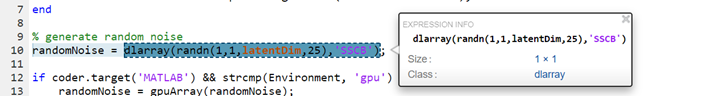## `dlarray` Limitations for Code Generation

### Recommended Usage

For code generation, use the `dlarray` (Deep Learning Toolbox) function to create deep learning arrays. For example, suppose you have a pretrained `dlnetwork` (Deep Learning Toolbox) network object in the `mynet.mat` MAT-file. To predict the responses for this network, create an entry-point function in MATLAB® as shown in this code.

```function a = foo(in) dlIn = dlarray(in, 'SSC'); persistent dlnet; if isempty(dlnet) dlnet = coder.loadDeepLearningNetwork('mynet.mat'); end dlA = predict(dlnet, dlIn); a = extractdata(dlA); end ```

### Using Variable-Size `dlarray`

You can generate code for MATLAB code that uses variable-size `dlarray` objects.

For example, define this MATLAB design file:

```function out = fooAdd(in1,in2) %#codegen dlIn1_1 = dlarray(in1); dlIn1_2 = dlarray(in2); out = dlIn1_1 + dlIn1_2; end```

Specify the two inputs `in1` and `in2` to be unbounded two-dimensional arrays of `single` type. Create the appropriate code configuration object `cfg` to generate generic C MEX code for `fooAdd`. Generate MEX code and run the generated MEX.

```t_in1 = coder.typeof(single(1),[inf inf],[1 1]); t_in2 = coder.typeof(single(1),[inf inf],[1 1]); codegen fooAdd -args {t_in1,t_in2} -report out = fooAdd_mex(single(eye(4,4)),single(ones(4,1)));```

When generating code for variable-size `dlarray` objects, adhere to these restrictions:

• The `U` dimension of a `dlarray` object must be of fixed size.

• If the `dlarray` data format `fmt` contains only one character, the corresponding data array `X` can have only one variable-size dimension. All other dimensions of `X` must be singleton.

• For operations between a `dlarray` object and a numeric array that might implicitly expand either operands, do not combine a fixed size `U` dimension of the `dlarray` object with a variable-size dimension of the numeric array.

• For unary operations such as `max`, `min`, and `mean` on a variable-size `dlarray` object, specify the intended working dimension explicitly as a constant value. See Automatic dimension restriction.

### Limitations

For deep learning arrays, code generation has the following limitations:

• The data format argument of the `dlarray` object must be a compile-time constant. For example,

```function out = foo() dlA = dlarray(ones(5,4),'SSC'); %fmt 'SSC' is constant . . . end```

• The code generation report does not display the size of the `dlarray` object. The size is always displayed as `1x1`.• In MATLAB, `dlarray` enforces the order of labels `'SCBTU'`. This enforcement eliminates ambiguous semantics in operations, which implicitly match labels between inputs. This behavior is mimicked during MEX code generation. However, for standalone code generation such as static, dynamic libraries, or executables, the data format follows the specification of the `fmt` argument of the `dlarray` object. As a result, if the input or output of an entry-point function is a `dlarray` object and its order of labels is not `'SCBTU'`, then the data layout will be different between the MATLAB environment and standalone code.

For example, consider a function `foo` with a `dlarray` object as an output.

```function dlA = foo() rng default dlA = dlarray(rand(5,4), 'BC'); end ```

In MATLAB, `dlA` is `4(C)`-by-`5(B)`.

```dlA = 4(C) × 5(B) dlarray 0.8147 0.9058 0.1270 0.9134 0.6324 0.0975 0.2785 0.5469 0.9575 0.9649 0.1576 0.9706 0.9572 0.4854 0.8003 0.1419 0.4218 0.9157 0.7922 0.9595 ```

For standalone code generation, `dlA` is `5(B)`-by-`4(C)`.

• For code generation, the `dlarray` input to the `predict` method of the `dlnetwork` object must be `single` data type.